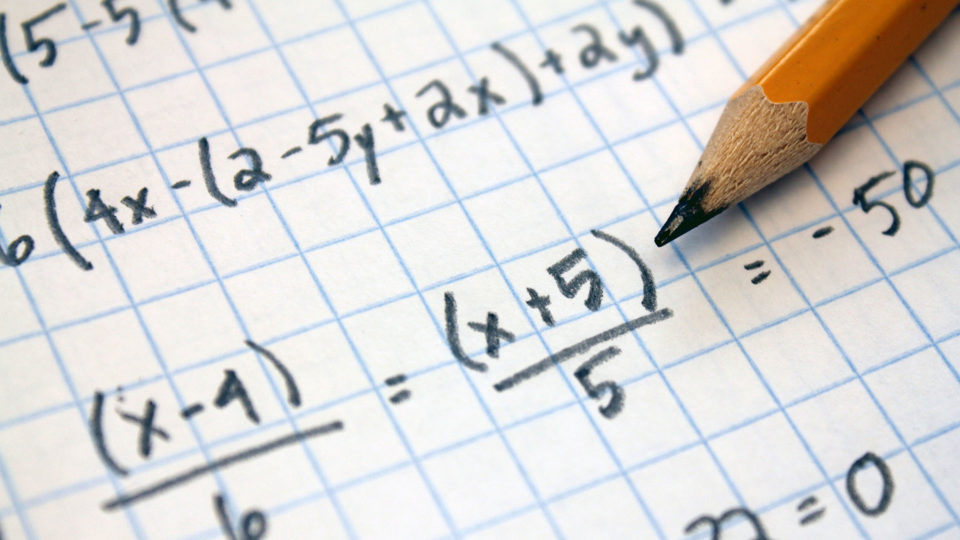# Calculations## 3 Pulley, belt drive and gear ratio

### Pulley ratios

• Below are videos of how to calculate gear ratios and speed calculation for a pair of gears and a compound gear set.

• The formula above applies to all drive systems. For pulleys, you would use the diameter instead of number of teeth.
• Chain and sprocket calculations will be the same as gear calculations.
• One thing to note, in gear drive systems, the gears will spin in opposite directions as they move through the gears.